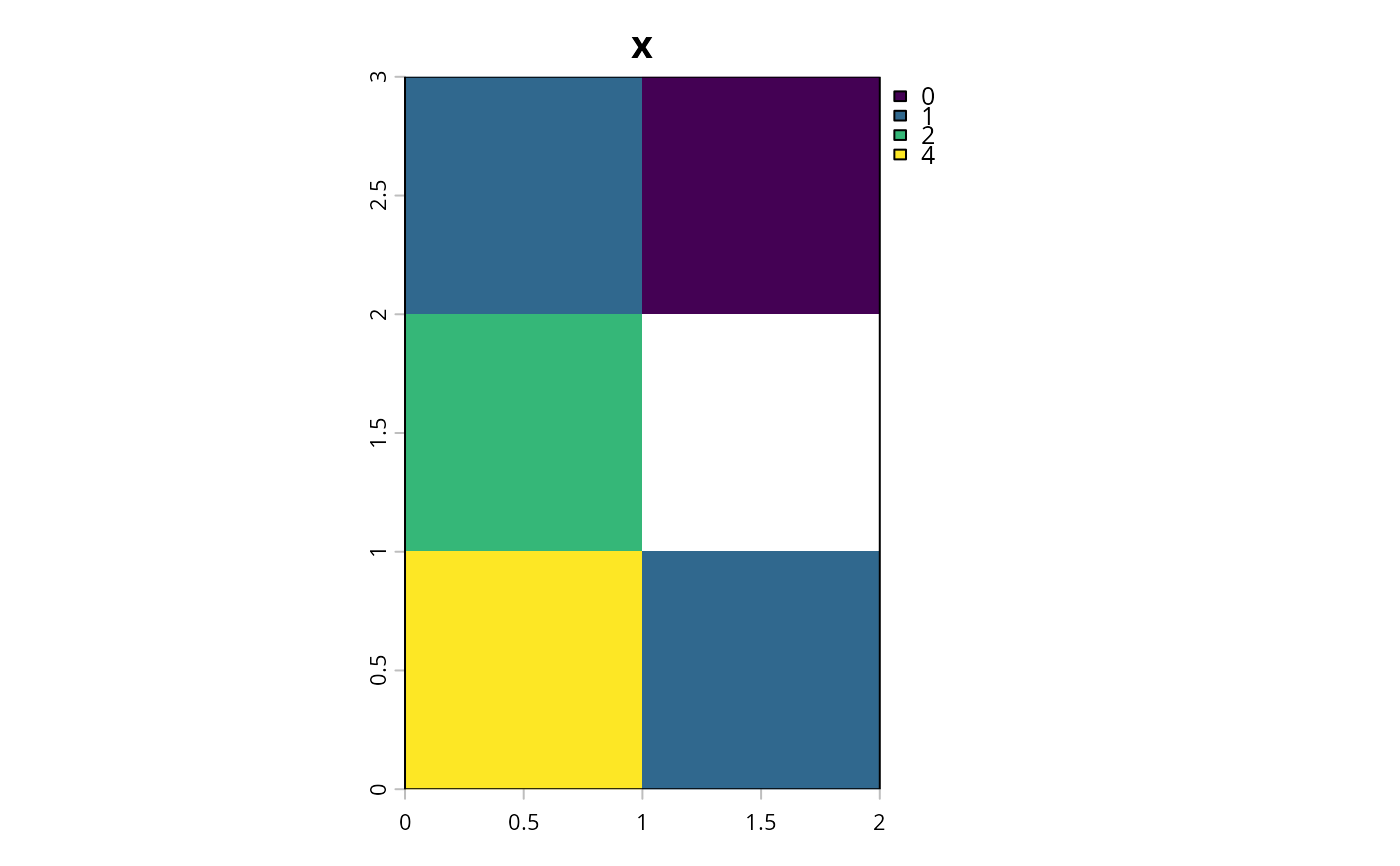Convert a RasterLayer object containing categorical identifiers into a RasterStack object where each layer corresponds to a different identifier and values indicate the presence/absence of that category in the input object.

binary_stack(x)

## Arguments

x

Raster object containing a single layer.

## Value

RasterStack object.

## Details

This function is provided to help manage data that encompass multiple management zones. For instance, this function may be helpful for preparing raster data for add_locked_in_constraints() and add_locked_out_constraints() since they require binary RasterStack objects as input arguments.

category_layer().

## Examples

# create raster with categorical identifers
x <- raster(matrix(c(1, 2, 3, 1, NA, 1), nrow = 3))

# convert to binary stack
y <- binary_stack(x)

# plot categorical raster and binary stack representation
# \dontrun{
plot(stack(x, y), main = c("x", "y[]", "y[]", "y[]"), nr = 1)# }